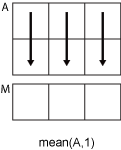# mean

## 语法

``M = mean(A)``
``M = mean(A,"all")``
``M = mean(A,dim)``
``M = mean(A,vecdim)``
``M = mean(___,outtype)``
``M = mean(___,missingflag)``

## 说明

``M = mean(A)` 返回 `A` 沿大小大于 1 的第一个数组维度的元素的均值。如果 `A` 是向量，则 `mean(A)` 返回元素均值。如果 `A` 为矩阵，那么 `mean(A)` 返回包含每列均值的行向量。如果 `A` 是多维数组，则 `mean(A)` 沿大小大于 1 的第一个数组维度计算，并将这些元素视为向量。此维度中 `M` 的大小变为 `1`，而所有其他维度的大小仍与在 `A` 中相同。如果 `A` 是表或时间表，则 `mean(A)` 返回包含每个变量的均值的单行表。 （自 R2023a 起）`

``M = mean(A,"all")` 返回 `A` 的所有元素的均值。`

``M = mean(A,dim)` 返回维度 `dim` 上的均值。例如，如果 `A` 为矩阵，则 `mean(A,2)` 返回包含每一行均值的列向量。`

``M = mean(A,vecdim)` 返回向量 `vecdim` 所指定的维度上的均值。例如，如果 `A` 是矩阵，则 `mean(A,[1 2])` 返回 `A` 中所有元素的均值，因为矩阵的每个元素包含在由维度 1 和 2 定义的数组切片中。`

``M = mean(___,outtype)` 返回上述任一语法的指定数据类型的均值。`outtype` 可以是 `"default"`、`"double"` 或 `"native"`。`

``M = mean(___,missingflag)` 指定包括还是忽略 `A` 中的缺失值。例如，`mean(A,"omitmissing")` 在计算均值时会忽略所有缺失值。默认情况下，`mean` 包括缺失值。`

## 示例

`A = [0 1 1; 2 3 2; 1 3 2; 4 2 2]`
```A = 4×3 0 1 1 2 3 2 1 3 2 4 2 2 ```
`M = mean(A)`
```M = 1×3 1.7500 2.2500 1.7500 ```

`A = [0 1 1; 2 3 2; 3 0 1; 1 2 3]`
```A = 4×3 0 1 1 2 3 2 3 0 1 1 2 3 ```
`M = mean(A,2)`
```M = 4×1 0.6667 2.3333 1.3333 2.0000 ```

```rng('default') A = randi(10,[4,2,3]); M = mean(A,2)```
```M = M(:,:,1) = 8.0000 5.5000 2.5000 8.0000 M(:,:,2) = 10.0000 7.5000 5.5000 6.0000 M(:,:,3) = 6.0000 5.5000 8.5000 10.0000 ```

```A(:,:,1) = [2 4; -2 1]; A(:,:,2) = [9 13; -5 7]; A(:,:,3) = [4 4; 8 -3]; M1 = mean(A,[1 2])```
```M1 = M1(:,:,1) = 1.2500 M1(:,:,2) = 6 M1(:,:,3) = 3.2500 ```

`M2 = mean(A,[1 2 3])`
```M2 = 3.5000 ```
`Mall = mean(A,"all")`
```Mall = 3.5000 ```

```A = single(ones(10,1)); M = mean(A,"native")```
```M = single 1 ```

`class(M)`
```ans = 'single' ```

`A = [1.77 -0.005 NaN -2.95; NaN 0.34 NaN 0.19]`
```A = 2×4 1.7700 -0.0050 NaN -2.9500 NaN 0.3400 NaN 0.1900 ```

`M = mean(A,"omitnan")`
```M = 1×4 1.7700 0.1675 NaN -1.3800 ```

## 输入参数

• 如果 `A` 是标量，则 `mean(A)` 返回 `A`

• 如果 `A` 为 0×0 空矩阵，则 `mean(A)` 返回 `NaN`

• `mean(A,1)` 计算 `A` 的每列中元素的均值，并返回一个 `1`×`n` 行向量。• `mean(A,2)` 计算 `A` 的每行中元素的均值，并返回一个 `m`×`1` 列向量。`dim` 大于 `ndims(A)` 或者 `size(A,dim)``1` 时，`mean` 返回 `A``outtype`输出数据类型
`"default"``double`，除非输入数据类型为 `single``duration``datetime``table``timetable`，在此情况下输出为 `"native"`
`"double"``double`，除非数据输入类型为 `duration``datetime``table``timetable`，在此情况下不支持 `"double"`
`"native"`

• 输入数据类型为 `logical`，在这种情况下，输出为 `double`

• 输入数据类型为 `char`，在这种情况下不支持 `"native"`

• 输入数据类型为 `timetable`，在这种情况下，输出为 `table`

`"includemissing"`所有支持的数据类型

`"includenan"``double`, `single`, `duration`
`"includenat"``datetime`
`"omitmissing"`所有支持的数据类型忽略 `A` 中的缺失值并基于较少的点计算均值。如果运算维度中的所有元素都缺失，则 `M` 中的对应元素也会缺失。
`"omitnan"``double`, `single`, `duration`
`"omitnat"``datetime`

## 详细信息

### 均值

`$\mu =\frac{1}{N}\sum _{i=1}^{N}{A}_{i}.$`